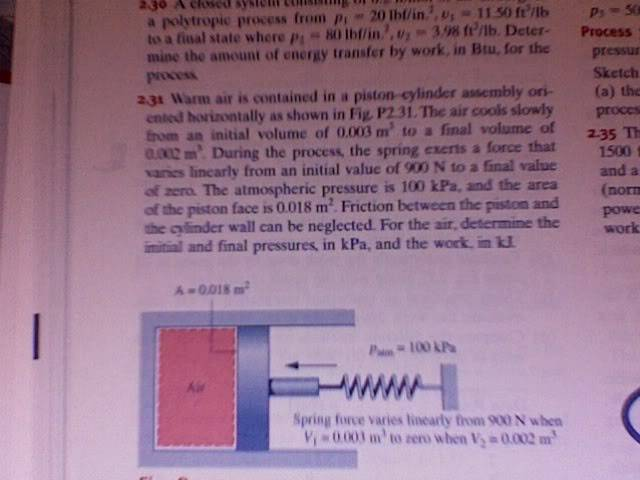# This Problem is Killing me and I don't know why!

## Homework Statement

It's 2.31Here is what I am thinking so far:

At V2 the system is at equilibrium, so p2 equals p_atm since the spring exerts no force.

So p2 is known.

I now need p1. I am not sure where to go from here. I have V1, V2 and p2.
I think that spring-force/area is part of p1...

Can someone give me a hint?Just a hint....

The work done by the piston on the air is

$$\int$$ P dV

You could write a linear equation for P as a fcn of V with the info you have, but of course geometrically this is just the area under the graph of P vs V, which in this case is trapezoidal in shape. Sketch the P-V trapezoid, calculate its area (watch the units), and you gave the work.

That sounds reasonable! But, let's look at it for a moment w/out the p-V diagram, I am confused as to how to right out $\int pdV$ What kind of compression is this considered? p is not constant, nor is V. I am just confused as to how to incorporate the spring force?

It says the spring force varies (decreases) linearly as the volume. You have two sets of (P,V) graph points, so you could write an equation of the form P = mV + c and use that to calculate the integral.

I wish I followed you. What is P=mV+c ? It say that the spring force varies linearly, not the pressure...Are you saying that p can be written as a function of volume since ot is just the sum of p_atm and F_spring/area?

That is that $$p(V)=F/A+p_{atm}$$ so I have to find out what F is...

So I need to write F_spring as a function of V somehow....

Last edited:
We know the following:

The spring force varies linearly from 9.00 kN to 0 kN. I'm writng this in kN for convenience, since kN / m^2 is kPa. Since the area of the piston is constant, and P = F / A, the pressure varies linearly also (as volume decreases) and so we can write a function P(V) = mV + c.

Now, when V = 0.003 m^3, F = 9.0 kN and P = (9.0 kN / 0.018 m^2) + 100 kPa = 600 kPa

When V = 0.002 m^3, F = 0.0 kN and P = (0.0 kN / 0.018 m^2) + 100 kPa = 100 kPa

Now using the two points (P,V) = (600,0.003), (100,0.002), we can find m and c for our function P(V).